## Illustrating geometric graphs

Oliver Knill, 12/27, 2014
The pictures from this article are about the chromatology of discrete surfaces, graphs for which every unit circle is a circular graph or discrete manifolds of dimension d which are defined in a recursively and graph theoretically. Unlike in most of the graph theory literature, we look at graphs as geometric objects which are powerful enough to model higher dimensional structures. Cohomology, homotopy or cobordism ideas look the same as in the continuum and become therefore powerful tools also in graph theory. Traditionally, one looks at graphs as one-dimensional simplicial complexes. But many ideas from the continuum go over almost verbatim by dropping this restriction. An overview. The use of higher dimensional topology in chromatic graph theory has been pioneered in the late 70ies by Steve Fisk. Researchers at that time used the concept of triangulations. The later is a difficult topic. Not only are there various inequivalent definitions and flavors of what a "triangulation" is, also seemingly obvious statements like that any topological manifold has a triangulation are simply wrong. To avoid any difficulty which origin from topology, we therefore almost stubbornly avoid any "Euclidean stuffing" notions like simplicial complex, CW-complexes and the like and remain in the discrete. Our approach becomes so completely graph theoretical. Therefore, the conjectures
 "Every surface has chromatic number 3,4 or 5" "Every sphere in dimension d has chromatic number d+1 or d+2"
are part of combinatorics and do not rely on any interpretation or definition used in topology. The definitions are simple and given in the preprint and below. As usual, G=(V,E) is always a finite simple graph, a graph without self-loops, multiple connections and having only finitely many vertices. Any subset A of the vertex set V generates a graph. For example, the unit sphere S(x) consisting of all vertices attached to x defines a graph in which all pairs (a,b) in S(x) are connected if (a,b) was in the edge set E.
 Let S-1=G-1 be the class of graphs which only contains the empty graph. Define inductively the set of geometric graphs Gd as the set of graphs for which S(x) is in Sd-1 or S(x) is in Bd-1 for all x in V. For G in Gd the boundary is the subgraph generated by δ G = S(x) in Bd-1. The graph int(G) = G - δ G is called the interior. The set of balls Bd in Gd is defined as the set of contractible graphs in Gd for which the boundary is in Sd-1. The set of spheres Sd in Gd is the set of non-contractible graphs for which removing an arbitrary vertex produces a graph in Bd.
These definitions make use of nontrivial facts we know to be true in the continuum like "homotopy spheres are spheres". The definitions were also first given here. Lets look at examples: G0 is the class of graphs without edges, B0 the class of graphs with one vertex and S0 the class of graphs with two vertices and no edges. Now, G1 is the class of graphs whose connectivity components are circular graphs with 4 or more elements, connected elements are in S1 and interval graphs are the graphs in B1. The octahedron or icosahedron are examples of graphs in S2 and wheel graphs are examples of graphs in B2. The 600 cell and 16 cell are examples of graphs in S3. Graphs G in Gd naturally define manifolds (embed the unit balls in Euclidean space and use this to build the charts of a compact topological manifold. The number of vertices is then the number of charts and the graph G is the "nerve graph".) A graph in G2 is called a surface. From the classical classification of surfaces done in the 19th century follows that orientability and genus defines the topological structure in G2. Contractibibility is defined inductively, where again S(x) is the unit sphere and B(x) the unit ball graph.
 A graph G is contractible if there is a vertex for which both S(x) and G - B(x) are contractible. The induction assumption is based on the statement that the empty graph is assumed to be contractible.
Contraction and its inverse, expansion steps generate a homotopy which is equivalent to the homotopy we know in the continuum. Geometric graphs in Gd have dimension d, where dimension is defined inductively:
 The empty graph has dimension -1. Define dim(G) = 1 + E[dim(S(x)], where E[X] is the expectation of a random variable X on the vertex set equipped with the counting measure.
As far as we know, this inductive dimension has first been defined in 2010. It is motivated by Menger-Uryson. Note however that the original Menger-Uryson dimension of a graph is 0 as the natural topology on the graph produces the discrete topology where every subset is open and closed. Also different is that the dimension of a graph can naturally become fractal. The notion of topology and homeomorphism has to be defined differently in order to achieve a "rubber geometry type" equivalence relation which for example assures that all cyclic graphs with 3 or more vertices are homeomorphic. Homotopy alone does not work as homotopy does not honor dimension. It is dimension and homotopy together which does the trick. In the following pictures, click on the thumbnail to get a high resolution picture.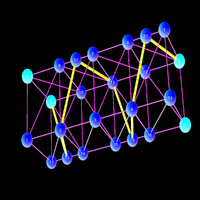A Fisk graph: a geometric surface with chromatic number 5. The embedding story suggests that 5 is the upper limit for all surfaces. (Like one can embed a one dimensional closed curve into the boundary of a three dimensional ball so that the complement in the open ball remains simply connected, it is possible to write an orientable surface into the three sphere bounding a four dimensional ball in such a way that the open inside of the later remains simply connected. In the non-orientable case, the embedding has to leave the 3 sphere at some places but we can keep the complement in the open four dimensional ball simply connected). To construct an example of the torus with chromatic number 5, start with a flat triangulated torus and cut rhomboids to get a geodesic with winding number (2,1). Now cut the torus along a circle and Dehn twist it so that the geodesic is broken. Now two adjacent vertices have odd degrees. It was Fisk who noticed that this leads to chromatic number 5. The 16 cell is the smallest three dimensional sphere. It is one of the 6 platonic solids in 4 dimensions, the analogue of the octahedron. Its dual is the tesseract. It can be colored with 4 colors which is the minimal number of colors for three dimensional spheres. We believe that any 3-sphere can be colored with 4 or 5 colors.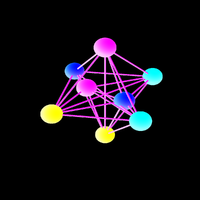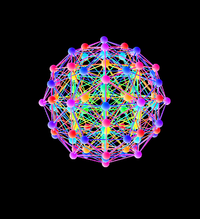The 600 cell is the largest three dimensional sphere which is also a platonic solid. Its dual is the 120-cell. The 600 cell needs 5 colors because of odd degree edges (actually all are odd). We believe any 3D sphere can be colored with 4 or 5 colors. Cutting three dimensional graphs: this is a screen shot from a game of cutting three dimensional graphs. This particular example is a self-cobordism G=G of a planar graph G with itself. Solving the game is to make all interior edges have even degree. If this can be achieved, the boundary graph is colored with 4 colors.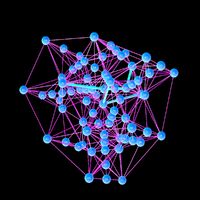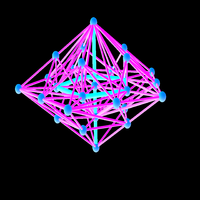The edges of odd degree are a union of closed curves (possibly ending at the boundary). If the host graph H of the cobordism is simply connected then they are the boundary of a surface. This is the reason why we are confident that one can always win the cutting game leading to chromatic number 4 for all planar graphs. Cutting an edge has the effect that the boundary of a wheel graph surface switches the parity of the edge degree. The game is to switch of all edge degree edges but unlike in On-Off games, the task can become more complicated as time goes on as the cutting increases the number of edges.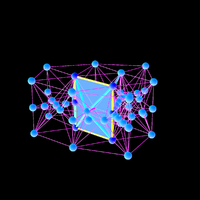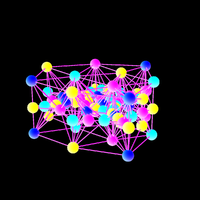The final frame of the cutting game. All interior edges are now even and the entire graph, including the boundary is now colored. There are various refinement steps possible. We used here only edge cuttings. An example of a cobordism between the octahedron and icosahedron. The odd degree interior edges are red, the others green.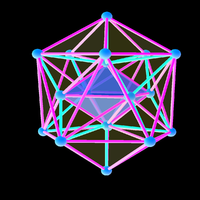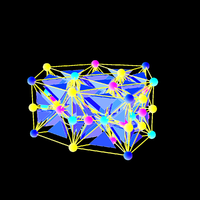If all the interior edges are even, then one can start with one tetrahedron, color it with 4 colors, then color all neighbors etc. The edge condition assures that no collisions occur. There are 4 Catalan solids which are spheres, the Disdyakis-Triacontahedron the Disdyakis-Dodecahedron, the Pentakis-Dodecahedron and Tetrakis Hexahedron. This is the Disdyakis Triacontahedron. It is 3 colorable.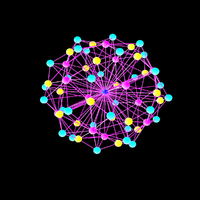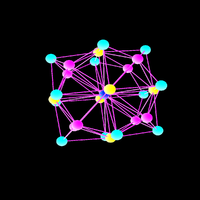This is the Disdyakis Dodecahedron. It is a two dimensional sphere which is 3 colorable. It has been realized in the 70ies already that a 3 colorable graph leads to a 4 colorable stellated graph. It is an obvious observation. What seems not have been realized yet before that one can use the idea to attack the 4 color theorem by solving a cutting game. This is the Pentakis-Dodecahedron an other two dimensional sphere which is 3 colorable.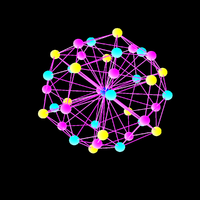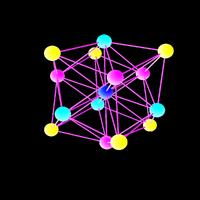This is the Tetrakis Hexahedron. The capped cube. It is 3 colorable. The idea of cobordism goes back to Poincare at the beginning of the 20'th century. It has become an important tool in topology. In discrete mathematics, it was Fisk who has experimented first with the notion but not in graph theory. The notion makes sense in graph theory if one looks at graphs as objects of arbitrary dimension and giving first a notion of dimension which corresponds to the notion we know in the continuum.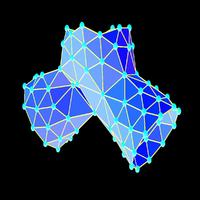Document history:
• 12/27/2014, first version
• 1/3/2015, adaptation of definition of Bd (skip definition for d=-1). See blog.